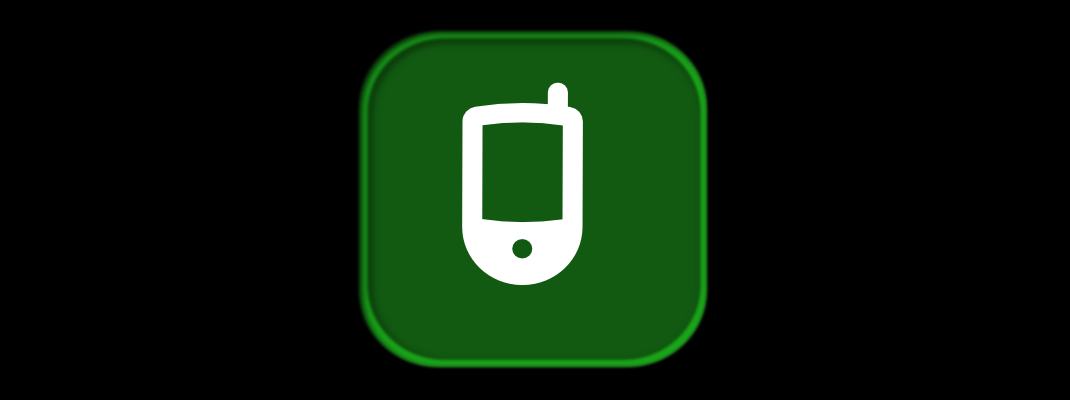## My mobile number is a prime number

My personal mobile number 9565804301 is a prime number. What is a prime number?\$ Any integer p greater than 1 is called a prime number if and only if its positive factors are 1 and the number p itself. In other words, the natural numbers which are completely divisible by 1 and themselves only and have no other factors, are called prime numbers. 2\$ ,\$ 3\$…

## Euler’s (Prime to) Prime Generating Equation

The greatest number theorist in mathematical universe, Leonhard Euler had discovered some formulas and relations in number theory, which were based on practices and were correct to limited extent but still stun the mathematicians. The prime generating equation by Euler is a very specific binomial equation on prime numbers and yields more primes than any other relations out there in…

## Numbers – The Basic Introduction

If mathematics was a language, logic was the grammar, numbers should have been the alphabet. There are many types of numbers we use in mathematics, but at a broader aspect we may categorize them in two categories: 1. Countable Numbers 2. Uncountable Numbers The numbers which can be counted in nature are called Countable Numbers and the numbers which can…

## Fermat Numbers

Fermat Number, a class of numbers, is an integer of the form \$ F_n=2^{2^n} +1 \ \ n \ge 0\$ . For example: Putting \$ n := 0,1,2 \ldots\$ in \$ F_n=2^{2^n}\$ we get \$ F_0=3\$ , \$ F_1=5\$ , \$ F_2=17\$ , \$ F_3=257\$ etc. Fermat observed that all the integers \$ F_0, F_1, F_2, F_3, \ldots\$ were prime…